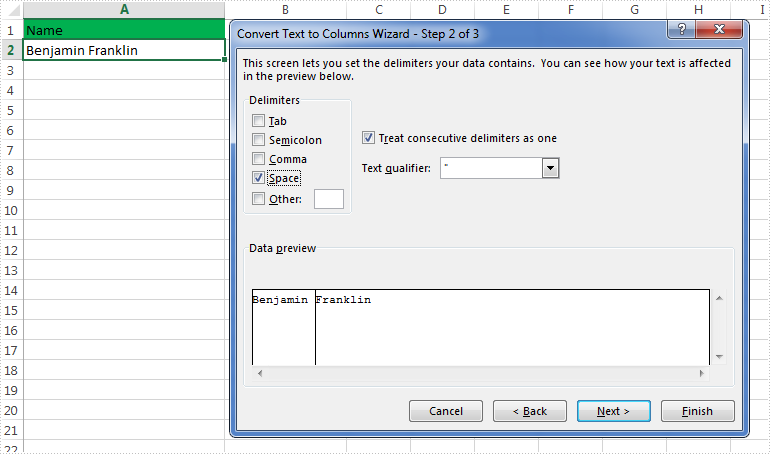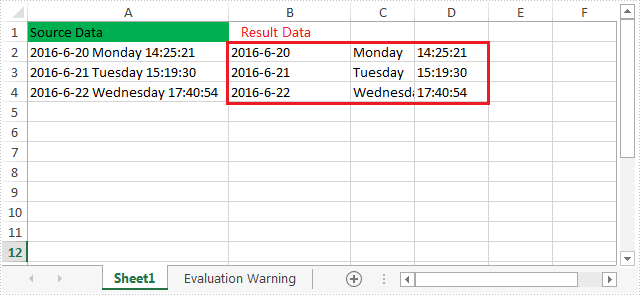# How to Split Excel Data into Multiple Columns in C#, VB.NET

It's a straightforward task to split data into multiple columns in Microsoft Excel, as we can use the Convert Text to Columns Wizard to achieve this feature easily, for example, split a column of names into a column of first name and a column of last name. Below picture shows how we can split data in Excel:In this article, we will introduce how to split excel data into multiple columns programmatically in C# and VB.NET using Spire.XLS for .NET.

Detail steps and code snippets:

Step 1: Create a new object of Workbook class and load the excel file.

```Workbook book = new Workbook();
```

Step 2: Get the first worksheet.

```Worksheet sheet = book.Worksheets;
```

Step 3: Split data into separate columns by the delimited characters – space.

Initialize a string and a string array, loop through from the second row to the last row, and split the data by the delimited characters – space, save the split data into the array and write the array items into separate columns of the first worksheet.

```string[] splitText = null;
string text = null;
for (int i = 1; i < sheet.LastRow; i++)
{
text = sheet.Range[i + 1, 1].Text;
splitText = text.Split(' ');
for (int j = 0; j < splitText.Length; j++)
{
sheet.Range[i + 1, 1 + j + 1].Text = splitText[j];
}
}
```

Step 4: Save the file.

```book.SaveToFile("result.xlsx", ExcelVersion.Version2010);
```

Effective screenshot:Full codes:

[C#]
```using Spire.Xls;

namespace Split_Data_into_Multiple_Columns
{
class Program
{
static void Main(string[] args)
{
Workbook book = new Workbook();
Worksheet sheet = book.Worksheets;
string[] splitText = null;
string text = null;
for (int i = 1; i < sheet.LastRow; i++)
{
text = sheet.Range[i + 1, 1].Text;
splitText = text.Split(' ');
for (int j = 0; j < splitText.Length; j++)
{
sheet.Range[i + 1, 1 + j + 1].Text = splitText[j];
}
}
book.SaveToFile("result.xlsx", ExcelVersion.Version2010);
}
}
}
```
[VB.NET]
```Imports Spire.Xls

Namespace Split_Data_into_Multiple_Columns
Class Program
Private Shared Sub Main(args As String())
Dim book As New Workbook()
Dim sheet As Worksheet = book.Worksheets(0)
Dim splitText As String() = Nothing
Dim text As String = Nothing
For i As Integer = 1 To sheet.LastRow - 1
text = sheet.Range(i + 1, 1).Text
splitText = text.Split(" "C)
For j As Integer = 0 To splitText.Length - 1
sheet.Range(i + 1, 1 + j + 1).Text = splitText(j)
Next
Next
book.SaveToFile("result.xlsx", ExcelVersion.Version2010)
End Sub
End Class
End Namespace
```